# Learning a fair loss function in pytorch

Most of the time when we are talking about deep learning, we are discussing really complicated architectures – essentially complicated sets of (mostly linear) equations. A second innovation in the application of deep learning though is the use of back propagation to fit under-determined systems. Basically you can feed these systems fairly complicated loss functions, and they will chug along with no problem. See a prior blog post of mine of creating simple regression weights for example.

Recently in the NIJ challenge, I used pytorch and the non-linear fairness function defined by NIJ. In the end me and Gio used an XGBoost model, because the data actually do not have very large differences in false positive rates between racial groups. I figured I would share my example here though for illustration of using pytorch to learn a complicated loss function that has fairness constraints. And here is a link to the data and code to follow along if you want.

First, in text math the NIJ fairness function was calculated as `(1 - BS)*(1 - FP_diff)`, where BS is the Brier Score and FP_diff is the absolute difference in false positive rates between the two groups. My pytorch code to create this loss function looks like this (see the `pytorch_mods.py` file):

``````# brier score loss function with fairness constraint
def brier_fair(pred,obs,minority,thresh=0.5):
bs = ((pred - obs)**2).mean()
over = 1*(pred > thresh)
majority = 1*(minority == 0)
fp = 1*over*(obs == 0)
min_tot = (over*minority).sum().clamp(1)
maj_tot = (over*majority).sum().clamp(1)
min_fp = (fp*minority).sum()
maj_fp = (fp*majority).sum()
min_rate = min_fp/min_tot
maj_rate = maj_fp/maj_tot
diff_rate = torch.abs(min_rate - maj_rate)
fin_score = (1 - bs)*(1 - diff_rate)
return -fin_score``````

I have my functions all stashed in different py files. But here is an example of loading up all my functions, and fitting my pytorch model to the training recidivism data. Here I set the threshold to 25% instead of 50% like the NIJ competition. Overall the model is very similar to a linear regression model.

``````import data_prep as dp
import fairness_funcs as ff
import pytorch_mods as pt

# Get the train/test data
train, test = dp.get_y1_data()
y_var = 'Recidivism_Arrest_Year1'
min_var = 'Race' # 0 is White, 1 is Black
x_vars = list(train)
x_vars.remove(y_var)

# Model learning fair loss function
m2 = pt.pytorchLogit(loss='brier_fair',activate='ident',
minority=min_var,threshold=0.25)
m2.fit(train[x_vars],train[y_var])``````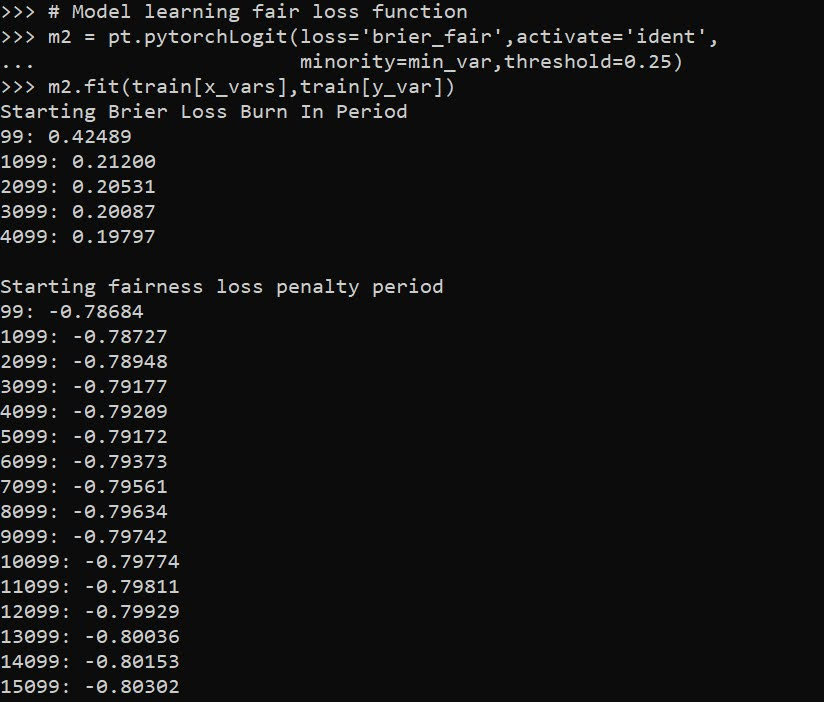I have a burn in start to get good initial parameter estimates with a more normal loss function before going into the more complicated function. Another approach would be to initialize the weights to the solution for a linear regression equation though. After that burn in though it goes into the NIJ defined fairness loss function.

Now I have functions to see how the different model metrics in whatever sample. Here you can see the model is quite balanced in terms of false positive rates in the training sample:

``````# Seeing training sample fairness
m2pp = m2.predict_proba(train[x_vars])[:,1]
ff.fairness_metric(m2pp,train[y_var],train[min_var],thresh=0.25)``````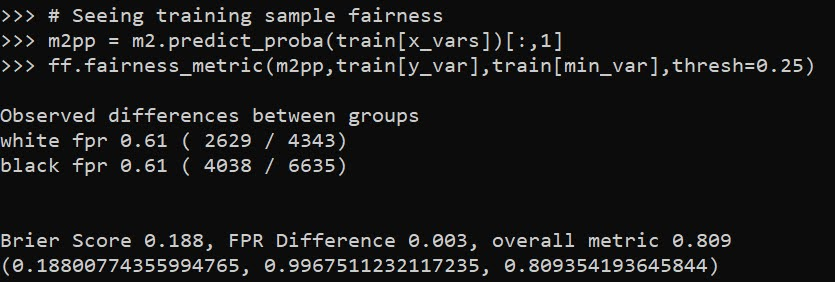But of course in the out of sample test data it is not perfectly balanced. In general you won’t be able to ensure perfect balance in whatever fairness metrics out of sample.

``````# Seeing test sample fairness
m2pp = m2.predict_proba(test[x_vars])[:,1]
ff.fairness_metric(m2pp,test[y_var],test[min_var],thresh=0.25)``````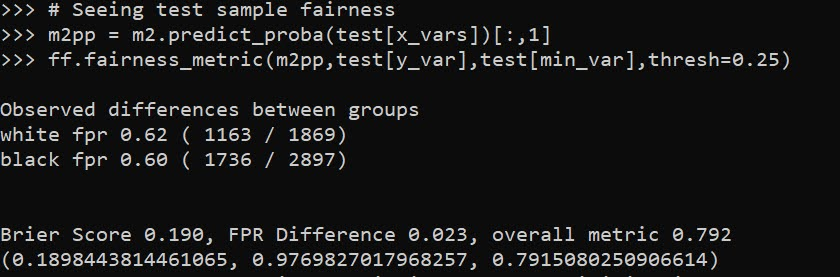It actually ends up that the difference in false positive rates between the two racial groups, even in models that do not incorporate the fairness constraint in the loss function, are quite similar. Here is a model using the same architecture but just the usual Brier Score loss. (Code not shown, see the `m1` model in the `01_AnalysisFair.py` file in the shared dropbox link earlier.)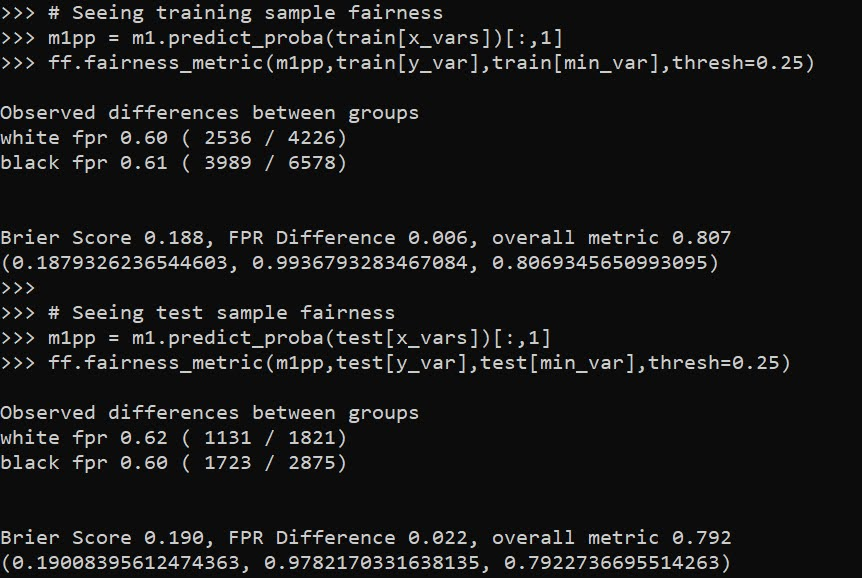You can read mine and Gio’s paper (or George Mohler and Mike Porter’s paper) about why this particular fairness function is not the greatest. In general it probably makes more sense to use an additive fairness loss instead of multiplicative, but in this dataset it won’t matter very much no matter how you slice it in terms of false positive rates. (It appears in retrospect the Compas data that started the whole false positive thing is somewhat idiosyncratic.)

There are other potential oddities that can occur with such fair loss functions. For example if you had the choice between false positive rates for `(white,black)` of `(0.62,0.60)` vs `(0.62,0.62)`, the latter is more fair, but the minority class is worse off. It may make more sense to have an error metric that sets the max false positive rate you want, and then just has weights for different groups to push them down to that set threshold.

These aren’t damning critiques of fair loss functions (these can be amended/changed to behave better), but in the end defining fair loss functions will be very tricky. Both for empirical reasons as well as for ethical ones – they will ultimately involve quite a few trade-offs.

# Fitting a pytorch model

Out of the box when fitting pytorch models we typically run through a manual loop. So typically something like this:

``````# Example fitting a pytorch model
# mod is the pytorch model object
crit = torch.nn.MSELoss(reduction='mean')
for t in range(20000):
y_pred = mod(x)   #x is tensor of independent vars
loss = crit(y_pred,y) #y is tensor of outcomes
loss.backward()
opt.step()``````

And this would use backpropogation to adjust our model parameters to minimize the loss function, here just the mean square error, over 20,000 iterations. Best practices are to both evaluate the loss in-sample and wait for it to flatten out, as well as evaluate out of sample.

I recently wrote some example code to make this process somewhat more like the sklearn approach, where you instantiate an initial model object, and then use a `mod.fit(X, y)` function call to fit the pytorch model. For an example use case I will just use a prior Compas recidivism data I have used for past examples on the blog (see ROC/Calibration plots, and Balancing False Positives). Here is the prepped CSV file to download to follow along.

So first, I load the libraries and then prep the recidivism data before I fit my predictive models.

``````###############################################
# Front end libraries/data prep

import pandas as pd
import numpy as np
import matplotlib.pyplot as plt
import torch

# Setting seeds
torch.manual_seed(10)
np.random.seed(10)

# Prepping the Compas data and making train/test

#Preparing the variables I want
recid_prep = recid[['Recid30','CompScore.1','CompScore.2','CompScore.3',
recid_prep['Male'] = 1*(recid['sex'] == "Male")
recid_prep['Fel'] = 1*(recid['c_charge_degree'] == "F")
recid_prep['Mis'] = 1*(recid['c_charge_degree'] == "M")
dum_race = pd.get_dummies(recid['race'])

# White for reference category
for d in list(dum_race):
if d != 'Caucasion':
recid_prep[d] = dum_race[d]

# reference category is separated/unknown/widowed
dum_mar = pd.get_dummies(recid['marital_status'])
recid_prep['Single'] = dum_mar['Single']
recid_prep['Married'] = dum_mar['Married'] + dum_mar['Significant Other']

#Now generating train and test set
recid_prep['Train'] = np.random.binomial(1,0.75,len(recid_prep))
recid_train = recid_prep[recid_prep['Train'] == 1].copy()
recid_test = recid_prep[recid_prep['Train'] == 0].copy()

#Independant variables
ind_vars = ['CompScore.1','CompScore.2','CompScore.3',
'African-American','Asian','Hispanic','Native American','Other',
'Single','Married']

# Dependent variable
y_var = 'Recid30'
###############################################``````

Now next part is more detailed, but it is the main point of the post. Typically we will make a pytorch model object something like this. Here I have various switches, such as the activation function (tanh or relu or pass in your own function), or the final function to limit predictions to 0/1 (either sigmoid or clamp or again pass in your own function).

``````# Initial pytorch model class
class logit_pytorch(torch.nn.Module):
def __init__(self, nvars, device, activate='relu', bias=True,
final='sigmoid'):
"""
Construct parameters for the coefficients
activate - either string ('relu' or 'tanh',
or pass in your own torch function
bias - whether to include bias (intercept) in model
final - use either 'sigmoid' to squash to probs, or 'clamp'
or pass in your own torch function
device - torch device to construct the tensors
default cuda:0 if available
"""
super(logit_pytorch, self).__init__()
# Creating the coefficient parameters
self.coef = torch.nn.Parameter(torch.rand((nvars,1),
device=device)/10)
# If no bias it is 0
if bias:
self.bias = torch.nn.Parameter(torch.zeros(1,
device=device))
else:
self.bias = torch.zeros(1, device=device)
# Various activation functions
if activate == 'relu':
self.trans = torch.nn.ReLU()
elif activate == 'tanh':
self.trans = torch.nn.Tanh()
else:
self.trans = activate
if final == 'sigmoid':
self.final = torch.nn.Sigmoid()
elif final == 'clamp':
# Defining my own clamp function
def tclamp(input):
self.final = tclamp
else:
# Can pass in your own function
self.final = final
def forward(self, x):
"""
predicted probability
"""
output = self.bias + torch.mm(x, self.trans(self.coef))
return self.final(output)``````

To use this though again we need to specify the number of coefficients to create, and then do a bunch of extras like the optimizer, and stepping through the function (like described at the beginning of the post). So here I have created a second class that behaves more like sklearn objects. I create the empty object, and only when I pass in data to the `.fit()` method it spins up the actual pytorch model with all its tensors of the correct dimensions.

``````# Creating a class to instantiate model to data and then fit
class pytorchLogit():
def __init__(self, loss='logit', iters=25001,
activate='relu', bias=True,
final='sigmoid', device='gpu',
printn=1000):
"""
loss - either string 'logit' or 'brier' or own pytorch function
iters - number of iterations to fit (default 25000)
activate - either string ('relu' or 'tanh',
or pass in your own torch function
bias - whether to include bias (intercept) in model
final - use either 'sigmoid' to squash to probs, or 'clamp'
or pass in your own torch function. Should not use clamp
with default logit loss
opt - ?optimizer? should add an option for this
device - torch device to construct the tensors
default cuda:0 if available
printn - how often to check the fit (default 1000 iters)
"""
super(pytorchLogit, self).__init__()
if loss == 'logit':
self.loss = torch.nn.BCELoss()
self.loss_name = 'logit'
elif loss == 'brier':
self.loss = torch.nn.MSELoss(reduction='mean')
self.loss_name = 'brier'
else:
self.loss = loss
self.loss_name = 'user defined function'
# Setting the torch device
if device == 'gpu':
try:
self.device = torch.device("cuda:0")
print(f'Torch device GPU defaults to cuda:0')
except:
print('Unsuccessful setting to GPU, defaulting to CPU')
self.device = torch.device("cpu")
elif device == 'cpu':
self.device = torch.device("cpu")
else:
self.device = device #can pass in whatever
self.iters = iters
self.mod = None
self.activate = activate
self.bias = bias
self.final = final
self.printn = printn
# Other stats to carry forward
self.loss_metrics = []
self.epoch = 0
def fit(self, X, y, outX=None, outY=None):
x_ten = torch.tensor(X.to_numpy(), dtype=torch.float,
device=self.device)
y_ten = torch.tensor(pd.DataFrame(y).to_numpy(), dtype=torch.float,
device=self.device)
# Only needed if you pass in an out of sample to check as well
if outX is not None:
x_out_ten = torch.tensor(outX.to_numpy(), dtype=torch.float,
device=self.device)
y_out_ten = torch.tensor(pd.DataFrame(outY).to_numpy(), dtype=torch.float,
device=self.device)
self.epoch += 1
# If mod is not already created, create a new one, else update prior
if self.mod is None:
loc_mod = logit_pytorch(nvars=X.shape, activate=self.activate,
bias=self.bias, final=self.final,
device=self.device)
self.mod = loc_mod
else:
loc_mod = self.mod
crit = self.loss
for t in range(self.iters):
y_pred = loc_mod(x_ten)
loss = crit(y_pred,y_ten)
if t % self.printn == 0:
if outX is not None:
pred_os = loc_mod(x_out_ten)
loss_os = crit(pred_os,y_out_ten)
res_tup = (self.epoch, t, loss.item(), loss_os.item())
print(f'{t}: insample {res_tup:.4f}, outsample {res_tup:.4f}')
else:
res_tup = (self.epoch, t, loss.item(), None)
print(f'{t}: insample {res_tup:.5f}')
self.loss_metrics.append(res_tup)
loss.backward()
opt.step()
def predict_proba(self, X):
x_ten = torch.tensor(X.to_numpy(), dtype=torch.float,
device=self.device)
res = self.mod(x_ten)
pp = res.cpu().detach().numpy()
return np.concatenate((1-pp,pp), axis=1)
def loss_stats(self, plot=True, select=0):
pd_stats = pd.DataFrame(self.loss_metrics, columns=['epoch','iteration',
'insamploss','outsamploss'])
if plot:
pd_stats2 = pd_stats.rename(columns={'insamploss':'In Sample Loss', 'outsamploss':'Out of Sample Loss'})
pd_stats2 = pd_stats2[pd_stats2['iteration'] > select].copy()
ax = pd_stats2[['iteration','In Sample Loss','Out of Sample Loss']].plot.line(x='iteration',
ylabel=f'{self.loss_name} loss')
plt.show()
return pd_stats``````

Again it allows you to pass in various extras, which here are just illustrations for binary predictions (like the loss function as the Brier score or the more typical log-loss). It also allows you to evaluate the fit for just in-sample, or for out of sample data as well. It also allows you to specify the number of iterations to fit.

So now that we have all that work done, here as some simple examples of its use.

``````# Creating a model and fitting
mod = pytorchLogit()
mod.fit(recid_train[ind_vars], recid_train[y_var])``````

So you can see that this is very similar now to sklearn functions. It will print at the console fit statistics over the iterations: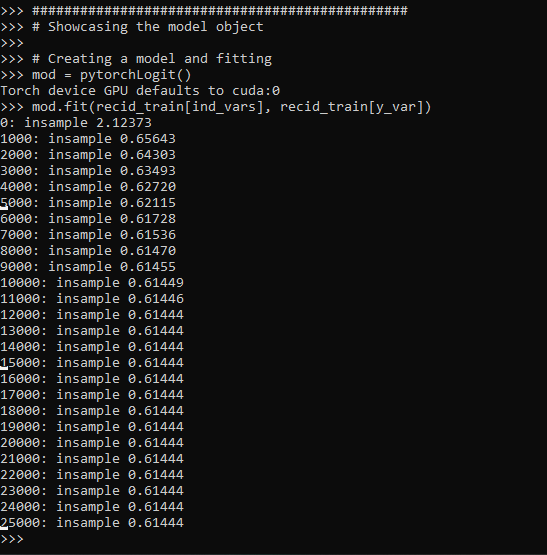So it defaults to 25k iterations, and you can see that it settles down much before that. I created a `predict_proba` function, same as most sklearn model objects for binary predictions:

``````# Predictions out of sample
predprobs = mod.predict_proba(recid_test[ind_vars])
predprobs # 1st column is probability 0, 2nd prob 1``````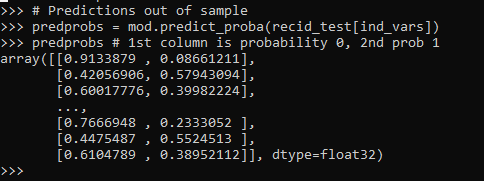And this returns a numpy array (not a pytorch tensor). Although you could modify to return a pytorch tensor if you wanted it to (or give an option to specify which).

Here is an example of evaluating out of sample fit as well, in addition to specifying a few more of the options.

``````# Evaluating predictions out of sample, more iterations
mod2 = pytorchLogit(activate='tanh', iters=40001, printn=100)
mod2.fit(recid_train[ind_vars], recid_train[y_var], recid_test[ind_vars], recid_test[y_var])``````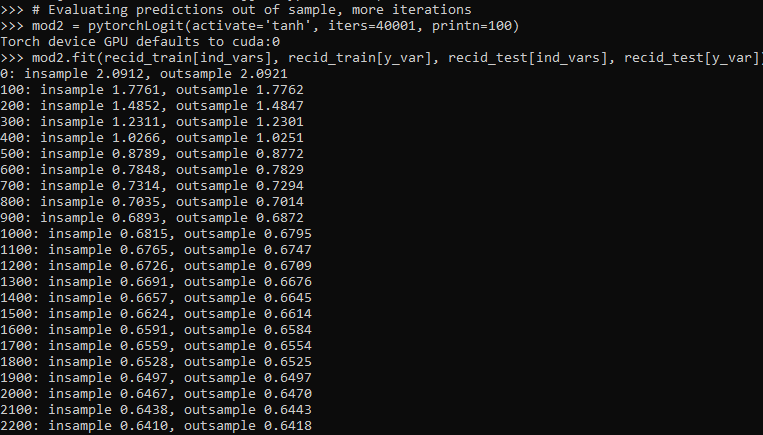I also have an object function, `.loss_stats()`, which gives a nice graph of in-sample vs out-of-sample loss metrics.

``````# Making a nice graph
dp = mod2.loss_stats()``````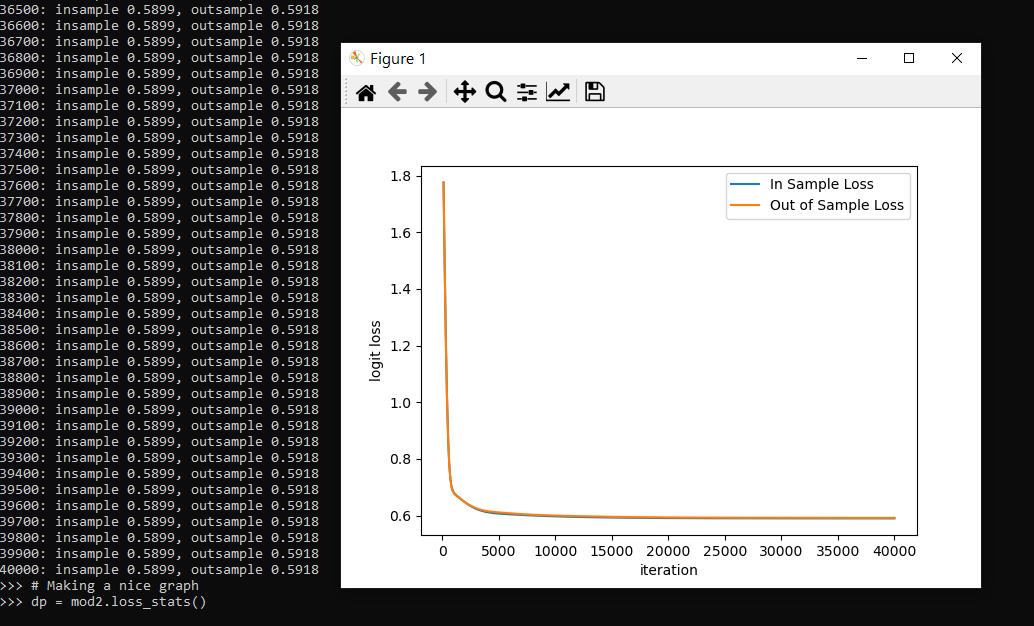We can also select the loss function to only show later iterations, so it is easier to zoom into the behavior.

``````# Checking out further along
mod2.loss_stats(select=10000)``````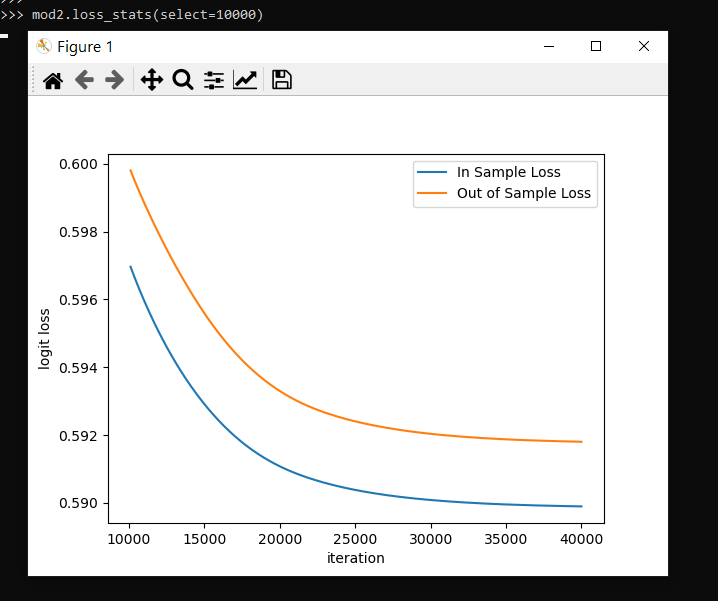And finally like I said you could modify some of your own functions here. So instead of any activation function I pass in the identity function – so this turns the model into something very similar to a vanilla logistic regression.

``````# Inserting in your own activation (here identity function)
def ident(input):
return input

mod3 = pytorchLogit(activate=ident, iters=40001, printn=2000)
mod3.fit(recid_train[ind_vars], recid_train[y_var], recid_test[ind_vars], recid_test[y_var])``````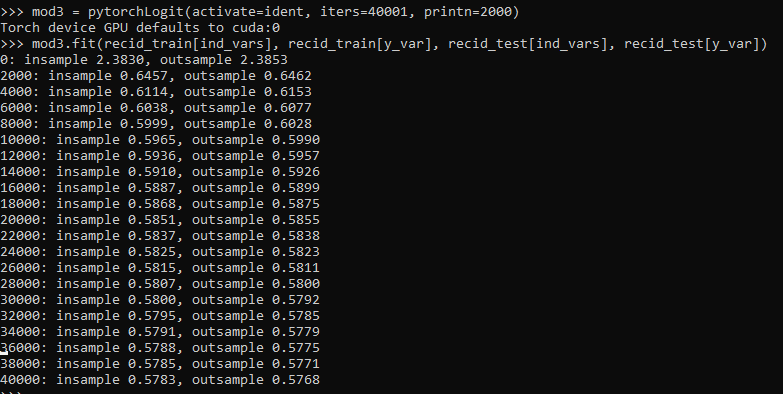And then if you want to access the coefficients weights, it is just going down the rabbit hole to the pytorch object:

``````# Can get the coefficients/intercept
print( mod3.mod.coef )
print( mod3.mod.bias )``````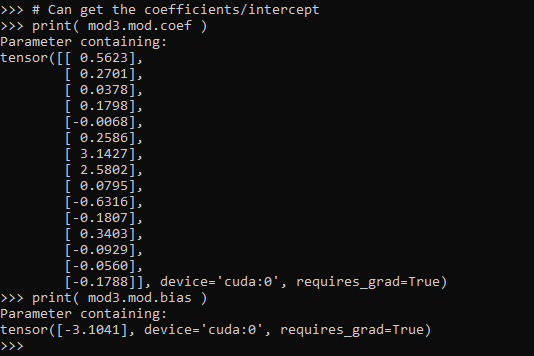This type of model can of course be extended however you want, but modifying the `pytorchLogit()` and `logit_pytorch` class objects to specify however detailed switches you want. E.g. you could specify adding in hidden layers.

One thing I am not 100% sure the best way to accomplish is loss functions that take more parameters, as well as the best way to set up the optimizer. Maybe use `*kwargs` for the loss function. So for my use cases I have stuffed extra objects into the initial class, so they are there later if I need them.

Also here I would need to think more about how to save the model to disk. The model is simple enough I could dump the tensors to numpy, and on loading re-do them as pytorch tensors.

# Regression with Simple Weights

I was reminded of this paper by Jung et al. on constructing simple rules via regression recently. So in the past few posts I have talked about how RTM (1,2) is aimed at making simple models. This is via variable selection and/or simplying the inputs to be binary yes/no. But in the end the final equation could be something like:

``log(Crime) = -0.56 + 0.6923*NearbyBars + 0.329*HighDensity311``

The paper linked above is about making the regression weights simple, so instead of a regression weight of 0.89728, you may just round the regression weight to 1. The Jung paper does a procedure where they use lasso regression and then round the weights. But there is a simpler approach IMO I will illustrate, just amend the lasso weights to push the coefficients to simple integers. (Also reminded by this example of using an iterative linear program to push weights to binary 0/1.)

So in lasso, you estimate your normal regression equation, but put a penalty on the weights that is typically something like `lambda*(sum(abs(reg_weights)) - 1)**2`. So if you have reg weights that add to more than 1, they are penalized by a particular amount (the lambda is a tuner to make the penalty higher/lower). And in the iterative algorithm to minimize your loss function plus this added penalty, it will converge to regression weights that meet the criteria of in total summing to around 1. Not exactly 1 but close.

You can however swap out that penalty term with whatever you want (or add to it additional penalties). I will show an example of using a penalty term to push regression coefficients towards integer values, creating simple regression weights.

# Why Simple Models?

Dan Simpson has a good blog post of the Jung paper and why simple models are sometimes preferable (and I also have a comment why simple models like this tend to work out well for CJ datasets). But here are few quick examples why you might want a simple model results.

Example 1: If you have people in the field who are tabulating data and making quick decisions, it may be they need to use pen/paper and make a quick decision. No time to input results into a computer and pop out a prediction. Imagine a nurse in the ER, or even your general practitioner. There may be quite a bit of utility in making a simple check list that says `if +4 on this scale, do a more intensive treatment`.

Example 2: You have a complicated, large database. It is easier to create a simple predictive model in SQL to serve up predictions (either because of latency or because of the complexity of the data pipeline). Instead of a complicated random forest, a linear regression with simple weights will be much easier to implement.

Example 3: Transparency. Complicated models are more difficult to understand and monitor. If you have a vested interest in presenting the model to outside parties, it may make sense to sacrifice some accuracy to make the model more interpretable. Also similar to lasso, I suspect these simple weights will reduce the variance of predictions.

The reason that these simple weights work well in practice for many social science examples you could interpret either in a good light or a bad one. For the half-empty interpretation, our models are not well identified – we can literally swap out various weights in our regression equation and get near similar predictions. So it is fools errand to try to find the regression equation that describes the underlying system. But you can flip that around as well, we don’t even need to find the perfect equation, we can identify quite a few good predictive equations. And why not pick a good equation that is easier to interpret?

# Pytorch Example

The example set of code here is very simple, so I will just put the python code entirely in this post. First I import my libraries I am using and change my directory.

``````############################################
import os
import torch
import statsmodels.api as sm
import statsmodels.formula.api as smf
import pandas as pd
import numpy as np

my_dir = r'D:\Dropbox\Dropbox\Documents\BLOG\regression_simpleweights\analysis'
os.chdir(my_dir)
############################################``````

Next I read in the data, which I have previously used as an example in prior blog posts on doctor visits for medicare patients. One thing to note here, is that I rescale the independent variables I am using to min/max. So the age variable instead of going from 65-90 like in the original data, now is scaled to be between 0/1. This is a problem intrinsic to lasso as well, in that you can change the scale of the input variables and it changes the weights. Here with the original data, the education variable has a tiny regression coefficient (0.2), but is highly stat significant. So without rescaling that variable, the model said to hell with your penalty and still converged to a solution of that regression weight is 0.2. If you divide the education variable by 5 though, the corresponding regression weight would change to around 1.

``````###########################################
#Data from Stata, https://www.stata-press.com/data/r16/gsem_mixture.dta
#see pg 501 https://www.stata.com/manuals/sem.pdf

y_dat = visit_dat[['drvisits']]
x_vars = ['private','medicaid','age','educ','actlim','chronic']
#rescaling variables to 0/1
x_dat = visit_dat[x_vars]
visit_dat[x_vars] = (x_dat - x_dat.min(axis=0)) / ( x_dat.max(axis=0)  - x_dat.min(axis=0) )
x_dat = visit_dat[x_vars] #intentional not a copy
###########################################``````

Now in the next part, I estimate the default linear regression model using statsmodels for reference. Then I stuff the results into pytorch tensors (which I will use later as default starting points for the pytorch estimates). Below is a pic of the resulting summary for the regression model (with the scaled variables, so is slightly different than my prior post).

``````###########################################
#Estimating the same model in statsmodel
#for confirmation of the result

stats_mod = smf.ols(formula='drvisits ~ private + medicaid + age + educ + actlim + chronic',
data=visit_dat)
sm_results = stats_mod.fit()
print(sm_results.summary())

#What is the mean squared error
pred = sm_results.get_prediction().summary_frame()
print( ((y_dat['drvisits'] - pred['mean'])**2).mean() )
#169513.0122252265 for sum
#46.10 for mean

#for setting default initial weights
coef_table = sm_results.params
###########################################``````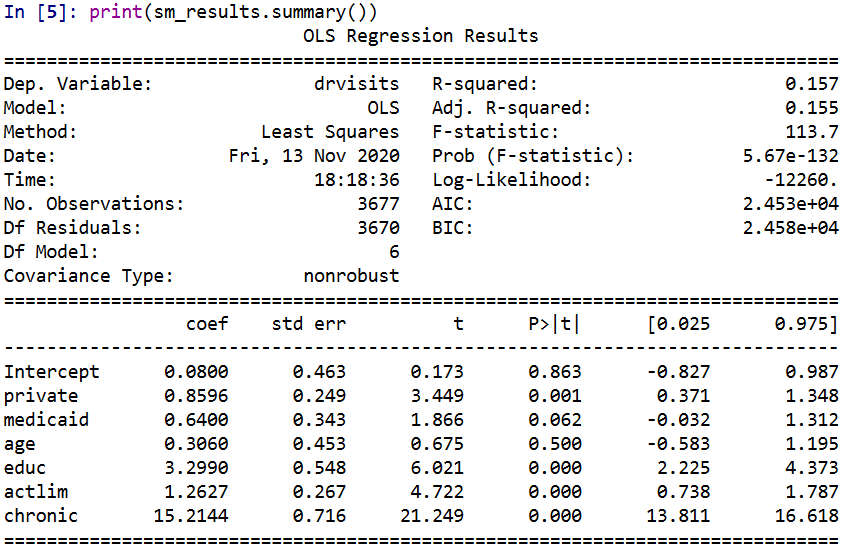Now creating the pytorch model is quite simple. For linear regression it is just one linear layer, and then setting the loss function to mean squared error. Then I create my own simple weight penalization factor in the `simp_loss` function. This takes the regression weights (not including the bias/intercept term), takes the difference between the observed weight and the rounded weight, takes the absolute value and sums those absolute values up. Then in the loop when I am fitting the model, you can see the `loss = criterion(y_pred, y_ten) + 0.4*simp_loss(model)` line. For the usual linear regression, it would just be the first criterion term. Here to add in the penalty term is super simple in pytorch, you just add it to the loss. (And you can incorporate additional penalities, the same way ala elastic-net. The Jung paper they put a penalty on the sum of coefficients as per the original lasso as well.)

Then the final part of the code after the loop is just putting the coefficients in a nicer data frame to print. And below the code snippet are the results.

``````###########################################
#Now estimating OLS model with simple coefficient
#Penalities in Pytorch

torch.manual_seed(10)

model = torch.nn.Sequential(
torch.nn.Linear(len(x_vars),1,bias=True)
)

##Initializing weights
#    model.weight = torch.nn.Parameter(coef_ten)
#    model.bias = torch.nn.Parameter(int_ten)

x_ten = torch.tensor( x_dat.to_numpy(), dtype=torch.float)
y_ten = torch.tensor( y_dat.to_numpy(), dtype=torch.float)

criterion = torch.nn.MSELoss(reduction='mean')

def simp_loss(mod):
dif = mod.weight - torch.round(mod.weight)
return dif.abs().sum()

for t in range(100000):
#Forward pass
y_pred = model(x_ten)
#Loss
loss = criterion(y_pred, y_ten) + 0.4*simp_loss(model)
if t % 1000 == 99:
print(f'iter: {t}, loss: {loss.item()}')
loss.backward()
optimizer.step()

#Making a nice dataframe of coefficients

coef_vars = ['Inter'] + x_vars
vals = list(model.bias.detach().numpy()) + list(model.weight.detach().numpy()[0,:])
res = pd.DataFrame(zip(coef_vars, vals), columns=['Var','Coef'])
print( res )
###########################################``````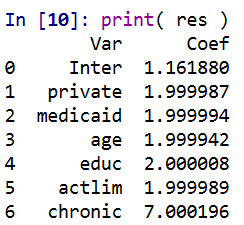Here I did not round the coefficients, so you can see that they are not exactly integer values, but are very close. So this will result in a lower loss than taking the usual linear regression coefficients and rounding them like in the noted Jung paper. It is a more direct approach. Also note that the intercept is not close to an integer value. I did not include the intercept in my penalty term. You could if you wanted to, but for most examples I don’t think it makes much sense to do that.

But one of the things that I have noticed playing around with pytorch more is that it is very difficult to get random initialized weights to converge to the same solution. That identification problem I mentioned earlier. One way is instead of using random initialized weights, is to initialize them to some reasonable values. If you uncomment the lines `with torch.no_grad():` in the above code and initialize the weights to start from the unregularized OLS solution, it converges much faster, has a slightly smaller mean square error term, and results in these effects: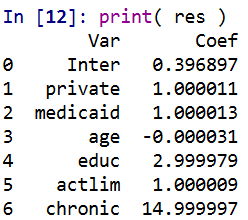So you can see in that solution it is exactly the same as rounding the initial OLS solution (ignoring the intercept again). But that may not always be the case. For example if actlim (activity limitations) and educ (education) had a very high correlation, it may be rounding both down is too big a hit to the fit of the equation, so one may go down and one go up. (You need to estimate the equation to know if things like that will occur.)

And that is all folks! While if I were sharing this more broadly, I would likely make a statsmodel like interface (and it appears they use cvxopt under the hood) instead of pytorch, it is very simple to amend pytorch to return simple weights, just add in the penalty to the loss function. Works the same way for lasso/ridge as it does for the simple weights example I give here.

Next up I want to try to figure out autograd in pytorch good enough to give standard errors for these various regression models I am estimating. While I don’t think hypothesis testing makes sense for these various models I am sharing, seeing a standard error that is very high may have prognostic value. In this case, if you had a very high standard error relative to the simple coefficient, it might suggest you should rescale the variable a different way or drop it entirely.

Also for this example, to be simple in the field it would not only need simple coefficients, but simple inputs as well. Wondering if there is a way to add in threshold layers in deep learning to automatically figure out the best way to make the inputs binary (e.g. above 70, educ below 10, etc.) instead of doing min/max scaling of the inputs.

# RTM Deep Learning Style

In my quest to better understand deep learning, I have attempted to replicate some basic models I am familiar with in criminology, just typical OLS and the more complicated group based trajectory models. Another example I will illustrate is doing a variant of Risk Terrain Modeling.

The typical way RTM is done is:

Data Prep Part:

1. create a set of independent variables for crime generators (e.g. bars, subway stops, liquor stores, etc.) that are either the distance to the nearest or the kernel density estimate
2. Turn these continuous estimates into dummy variables, e.g. if within 100 meters = 1, else = 0. For kernel density they typically z-score and if a z-score > 2 the dummy variable equals 1.
3. Do 2 for varying distance/bandwidth selections, e.g. 100 meters, 200 meters, etc. So you end up with a collection of distance variables, e.g. Bars_100, Bars_200, Bars_400, etc.

Modeling Part

1. Fit a Lasso regression predicting your crime outcome constraining all of the variables to be positive. (So RTM will never say a crime generator has a negative effect.)
2. For the variables that passed this Lasso stage, then do a variable selection routine. So instead of the final model having Bars_100 and Bars_400, it will only choose one of those variables.

For the modeling part, we can replicate various parts of these in a deep learning environment. For the constrain the coefficients to be positive, when you see folks refer to a “RelU” or the rectified linear unit function, all this means is that the coefficients are constrained to be positive. For the variable selection part, I needed to hack my own – it ends up being a combo of a custom dropout scheme and then pruning in deep learning lingo.

Although RTM is typically done on raster grid cells for the spatial unit of analysis, this is not a requirement. You can do all these steps on vector (e.g. street segments) or other areal spatial units of analysis.

Here I illustrate using street units (intersections and street segments) from DC. The crime generator data I take from my dissertation (and I have a few pubs in Crime & Delinquency based on that work). The crime data I illustrate using 2011 violent Part 1 UCR (homicide, agg assault, robbery, no rape in the public data).

The crime dataset is over time, and I describe in an analysis (with Billy Zakrzewski) on examining pre/post crime around DC medical marijuana dispensaries.

The data and code to replicate can be downloaded here. It is python, and for the deep learning model I used pytorch.

# RTM Example in Python

So I will walk through briefly my second script, `01_DeepLearningRTM.py`. The first script, `00_DataPrep.py`, does the data prep, so this data file already has the crime generator variables prepared in the manner RTM typically creates them. (The `rtm_dl_funcs.py` has the functions to do the feature extraction and create the deep learning model, to do distance/density in sci-kit is very slick, only a few lines of code.)

So first I just define the libraries I will be using, and import my custom rtm functions I created.

``````######################################################
import numpy as np
import pandas as pd
import torch
device = torch.device("cuda:0")
import os
import sys

my_dir = r'C:\Users\andre\OneDrive\Desktop\RTM_DeepLearning'
os.chdir(my_dir)
sys.path.append(my_dir)
import rtm_dl_funcs
######################################################``````

The next set of code grabs the crime data, and then defines my variable sets. I have plenty more crime generator data from my dissertation, but to make it easier on myself I just focus on distance to metro entrances, the density of 311 calls (a measure of disorder), and the distance and density of alcohol outlets (this includes bars/liquor stores/gas stations that sell beer, etc.).

Among these variable sets, the final selected model will only choose one within each set. But I have also included the ability for the model to incorporate other variables that will just enter in no-matter what (and are not constrained to be positive). This is mostly to incorporate an intercept into the regression equation, but here I also include the percent of sidewalk encompassing one of my street units (based on the Voronoi tessellation), and a dummy variable for whether the street unit is an intersection. (I also planned on included the area of the tessalation, but it ended up being an explosive effect, my dissertation shows its effect is highly non-linear, so didn’t want to worry about splines here for simplicity.)

``````######################################################
#Get the Prepped Data

#Variable sets for each
db = [50, 100, 200, 300, 400, 500, 600, 700, 800]
metro_set = ['met_dis_' + str(i) for i in db]
alc_set = ['alc_dis_' + str(i) for i in db]
alc_set += ['alc_kde_' + str(i) for i in db]
c311_set = ['c31_kde_' + str(i) for i in db]

#Creating a few other generic variables
crime_data['PercSidewalk'] = crime_data['SidewalkArea'] / crime_data['AreaMinWat']
crime_data['Const'] = 1
const_li = ['Const','Intersection','PercSidewalk']
full_set = const_li + alc_set + metro_set + c311_set
######################################################``````

The next set of code turns my data into a set of torch tensors, then I grab the size of my independent variable sets, which I will end up needing when initializing my pytorch model.

Then I set the seed (to be able to reproduce the results), create the model, and set the loss function and optimizer. I use a Poisson loss function (will need to figure out negative binomial another day).

``````######################################################
#Now creating the torch tensors
x_ten = torch.tensor(crime_data[full_set].to_numpy(), dtype=float)
y_ten = torch.tensor(crime_data['Viol_2011'].to_numpy(), dtype=float)
out_ten = torch.tensor(crime_data['Viol_2012'].to_numpy(), dtype=float)

#These I need to initialize the deep learning model
gen_lens = [len(alc_set), len(metro_set), len(c311_set)]

#Creating the model
torch.manual_seed(10)

model = rtm_dl_funcs.RTM_torch(const=len(const_li),
gen_list=gen_lens)
criterion = torch.nn.PoissonNLLLoss(log_input=True, reduction='mean')
print( model )
######################################################``````

If you look at the printed out model, it gives a nice summary of the different layers. We have our one layer for the fixed coefficients, and another three sets for our alcohol outlets, 311 calls, and metro entrances. We then have a final cancel layer. The idea behind the final cancel layer is that the variable selection routine in RTM can still end up not selecting any variables for a set. I ended up not using it here though, as it was too aggressive in this example. (So will need to tinker with that some more!)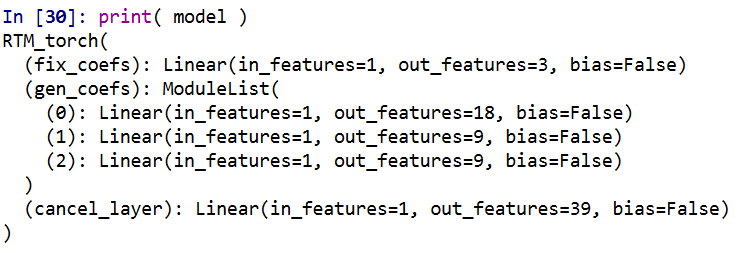The variable selection routine is very volatile – so if you have very correlated inputs, you can essentially swap one for the other and get near equivalent predictions. I often see folks who do RTM analyses say something along the lines of, “OK this RTM selected A, and this RTM selected B, so they are different effects in these two samples” (sometimes pre/post, other times comparing different areas, and other times different crime outcomes). I think this is probably wrong though to make that inference, as there is quite a bit of noise in the variable selection process (and the variable selection process itself precludes making inferences on the coefficients themselves).

My deep learning example inherited the same problems. So if you change the initialized weights, it may end up selecting totally different inputs in the end. To get the variable selection routine to at least select the same crime generator variables in my tests, I do a burn in period in which I implement a random dropout scheme. So instead of the typical dropout, for every forward pass it does a random dropout to only select one variable randomly out of each crime generator set. After that converges, I then use a pruning layer to only pick the coefficient that has the largest effect, and again do a large set of iterations to make sure the results converged. So different means but same ends to the typical RTM steps 4 and 5 above. I also have like I said a ReLU transformation after each layer, so the crime generator variables are always positive, any negative effects will be pruned out.

One thing that is nice about deep learning is that it can be quite fast. Here each of these 10,000 iteration sets take less than a minute on my desktop with a GPU. (I’ve been prototyping models with more parameters and more observations at work on my laptop with just a CPU that only take like 10 to 20 minutes).

``````######################################################
#Burn in part, random dropout
for t in range(10000):
#Forward pass
y_pred = model(x=x_ten)
#Loss
loss_insample = criterion(y_pred, y_ten)
loss_insample.backward(retain_graph=True)
optimizer.step()
if t % 1000 == 0:
print(f'loss: {loss_insample.item()}' )

#Switching to pruning all but the largest effects
model.l1_prune()

for t in range(10000):
#Forward pass
#Loss
loss_insample = criterion(y_pred, y_ten)
loss_insample.backward(retain_graph=True)
optimizer.step()
if t % 1000 == 0:
print(f'loss: {loss_insample.item()}' )

print( model.coef_df(nm_li=full_set, cancel=False) )
######################################################``````

And this prints out the results (as incident rate ratios), so you can see it selected 50 meters alcohol kernel density, 50 meters distance to the nearest metro station, and kernel density for 311 calls with an 800 meter bandwidth.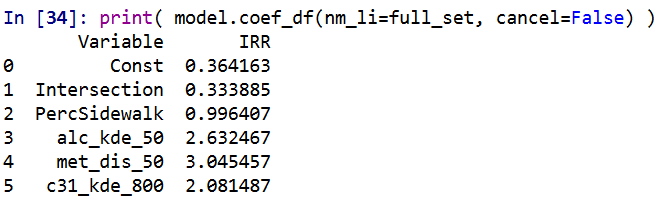I have in the code another example model when using a different seed. So testing out on around 5 different seeds it always selected these same distance/density variables, but the coefficients are slightly different each time. Here is an example from setting the seed to 12.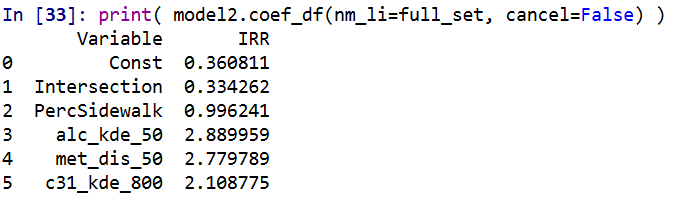These models are nothing to brag about, using the typical z-score the predictions and set the threshold to above 2, the PAI is only around 3 (both for in-sample 2011 and out of sample 2012 is slightly lower). It is a tough prediction task – the mean number of violent crimes per street unit per year is only 0.3. Violent crime is fortunately very rare!

But with only three different risk variables, we can do a quick conjunctive analysis, and look at the areas of overlap.

``````######################################################
#Adding model 1 predictions back into the dataset
crime_data['Pred_M1'] = pred_mod1

#Check out the areas of overlapping risk
mod1_coef = model.coef_df(nm_li=full_set, cancel=False)
risk_vars = list(set(mod1_coef['Variable']) - set(const_li))
conj_set = crime_data.groupby(risk_vars, as_index=False)['Const','Pred_M1','Viol_2012'].sum()
print(conj_set)
######################################################``````

In this table `Const` is the total number of street units selected, `Pred_M1` is the expected number of crimes via Model 1, and then I show how well it conforms to the predictions out of sample 2012. So you can see in the aggregate the predictions are not too far off. There only ends up being one street unit that overlaps for all three risk factors in the study area.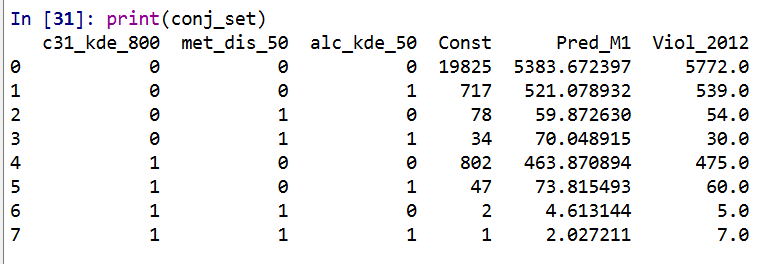I believe the predictions would be better if I included more crime generator variables. But ultimately the nature of how RTM works it trades off accuracy for simple models. Which is fair – it helps to ease the nature of how a police department (or some other entity) responds to the predictions.

But this trade off results in predictions that don’t fare as well compared with more complicated models. For example I show (with Wouter Steenbeek) that random forests do much better than RTM. To make those models more interpretable we did local decompositions for hot spots, so say this hot spot is 30% alcohol outlets, 20% nearby apartments, etc.

So there is no doubt more extensions for RTM you could do in a deep learning framework, but they will likely always result in more complicated and less interpretable models. Also here I don’t think this code will be better than the traditional RTM folks, the only major benefit of this code is it will run faster – minutes instead of overnight for most jobs.

# GPU go brrr: Estimating OLS (with standard errors) via deep learning

So a bunch of my criminologists friends have methods envy. So to help them out, I made some python functions to estimate OLS models using pytorch (a deep learning python library). So if you use my functions, you can just append something like an analysis with GPU accelerated deep learning to the title of your paper and you are good to go. So for example, if your paper is `An analysis of the effects of self control on asking non-questions at an ASC conference`, you can simply change it to `A GPU accelerated deep learning analysis of the effects of self control on asking non-questions at an ASC conference`. See how that works, instantly better.

Code and data saved here. There are quite a few tutorials on doing OLS in deep learning libraries, only thing special here is I also calculate the standard errors for OLS in the code as well.

# python code walkthrough

So first I just import the libraries I need. Then change the directory to wherever you saved the code on your local machine, and then import my `deep_ols` script. The `deep_ols` script also relies on the `scipy.stats` library, but besides pytorch it is the usual scientific python stack.

``````import os
import sys
import torch
import statsmodels.api as sm
import pandas as pd
import numpy as np

###########################################
#Setting the directory and importing
#My deep learning OLS function

my_dir = r'C:\Users\andre\OneDrive\Desktop\DeepLearning_OLS'
os.chdir(my_dir)
sys.path.append(my_dir)
import deep_ols
############################################``````

For the dataset I am using, it is a set of the number of doctor visits I took from some of the Stata docs.

``````#Data from Stata, https://www.stata-press.com/data/r16/gsem_mixture.dta
#see pg 501 https://www.stata.com/manuals/sem.pdf

visit_dat['intercept'] = 1
y_dat = visit_dat['drvisits']
x_dat = visit_dat[['intercept','private','medicaid','age','educ','actlim','chronic']]

print( y_dat.describe() )
print( x_dat.describe() )``````

Then to estimate the model it is simply below, the function returns the torch model object, the variance/covariance matrix of the coefficients (as a torch tensor), and then a nice pandas data frame of the results.

``mod, vc, res = deep_ols.ols_deep(y=y_dat,x=x_dat)``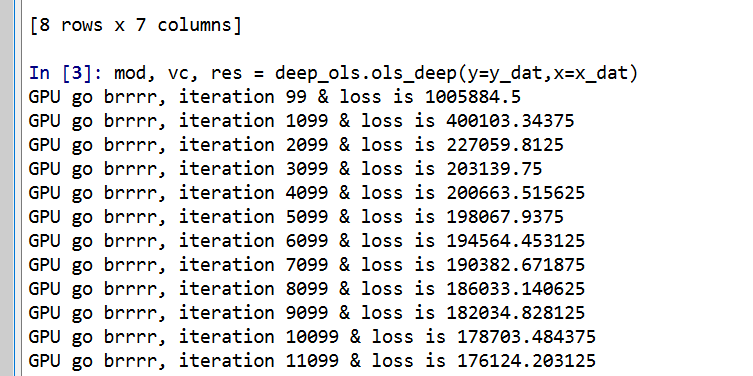As you can see, it prints out GPU accelerated loss results so fast for your pleasure.

Then, like a champ you can see the OLS results. P-values < 0.05 get a strong arm, those greater than this get a shruggie. No betas to be found in my model results.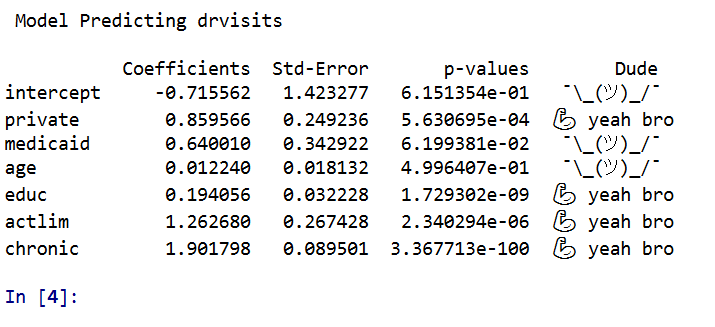Just to confirm, I show you get the same results using `statsmodel`.

``````stats_mod = sm.OLS(y_dat,x_dat)
sm_results = stats_mod.fit()
print(sm_results.summary())``````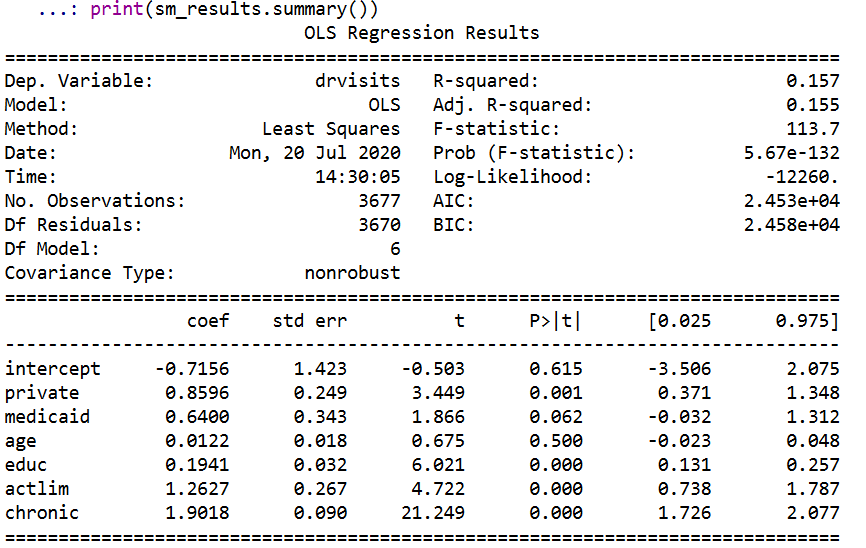So get on my level bro and start estimating your OLS models with a GPU.

# Some Notes

First, don’t actually use this in real life. I don’t do any pre-processing of the data, so if you have some data that is not on a reasonable scale (e.g. if you had a variable income going from 0 to \$500,000) all sorts of bad things can happen. Second, it is not really accelerated – I mean it is on the GPU and the GPU goes brr, but I’m pretty sure this will not ever be faster than typical OLS libraries. Third, this isn’t really “deep learning” – I don’t have any latent layers in the model.

Also note the standard errors for the coefficients are just calculated using a closed form solution that I pilfered from this reddit post. (If anyone has a textbook reference for that would appreciate it! I Maria Kondo’d most of my text books when I moved out of my UTD office.) If I figure out the right gradient calculations, I could probably also add ‘robust’ somewhere to my suggested title (by using the outer product gradient approach to get a robust variance/covariance matrix for the coefficients).

As a bonus in the data file, I have a python script that shows how layers in a vanilla deep learning model (with two layers) are synonymous with a particular partial least squares model. The ‘relu activation function’ is equivalent to a constraint that restricts the coefficients to be positive, but otherwise will produce equivalent predictions.

You could probably do some nonsense of saving the Jacobian matrix to get standard errors for the latent layers in the neural network if you really wanted to.

To end, for those who are really interested in deep learning models, I think a better analogy is that they are just a way to specify and estimate a set of simultaneous equations. Understanding the nature of those equations will help you relate how deep learning is similar to regression equations you are more familiar with. The neuron analogy is just nonsense IMO and should be retired.

# Using pytorch to estimate group based traj models

Deep learning, tensors, pytorch. Now that I have that seo junk out of the way 🙂 – I’ve been trying to teach myself some “Deep Learning”, as it is what all of the cool kids are doing these days.

I was having a hard time though with many of the different examples. Many are for image data, and so it was hard for me to translate that to actual applications I am interested in. Many do talk about dimension reduction and reducing to hidden layers, so I thought that was similar in nature to latent class analysis, such as group-based-trajectory-modelling (GBTM).

If you aren’t familiar with GBTM, imagine a scenario in which you cluster data, and then you estimate a different regression model to predict some outcome for each subset of the clustered data. This is just a way to do that whole set-up in one go, instead of doing each part separately. It has quite a few different names – latent class analysis and mixture modelling are two common ones. The only thing really different about GBTM is that you have repeated observations – so if you follow the same person over time, they should always be assigned to the same cluster/mixture.

In short you totally can do GBTM models in deep learning libraries (as I will show), but actually most examples that I have walked through are more akin to dimension reduction of columns (so like PCA/Canonical Correlation). But the deep learning libraries are flexible enough to do the latent class analysis I want here. As far as I can tell they are basically just a nice way to estimate systems of equations (with a ton of potential parameters, and do it on the GPU if you want).

So I took it as a challenge to estimate GBTM models in a deep learning library – here pytorch. In terms of the different architectures/libraries (e.g. pytorch, tensorflow, Vowpal Wabbit) I just chose pytorch because one of my co-workers suggested pytorch was easier to learn!

I’ve posted a more detailed notebook of the code, but it worked out quite well. So first I simulated two groups of data (50 observations in each group and 11 time periods). I added a tiny bit of random noise, so this (I was hoping) should be a pretty tame problem for the machine to learn.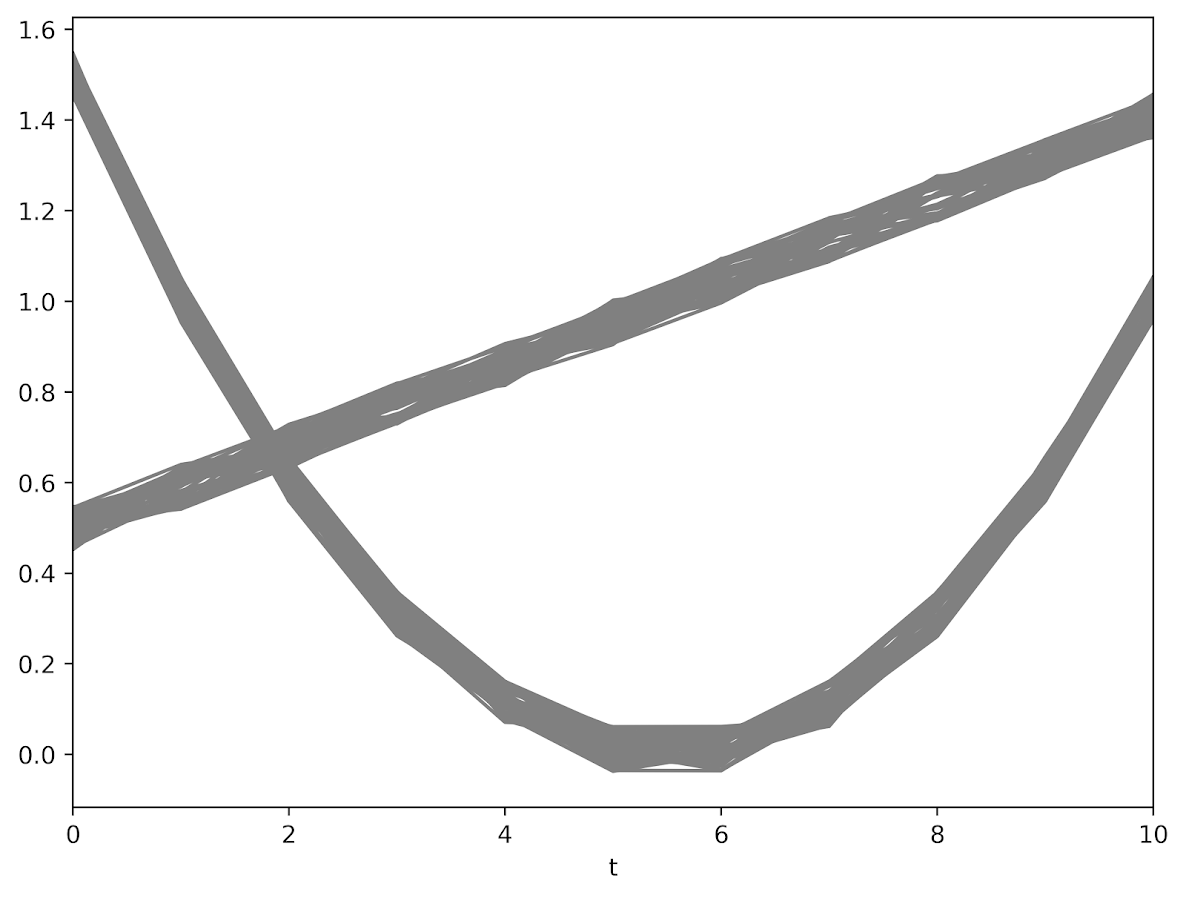The code to generate a pytorch module and have the machine churn out the gradients is pretty slick (less than 30 lines total of non-comments). Many GBTM code bases make you do the analysis in wide format (so one row is an observation), but here I was able to figure out how to set it up in long data format, which makes it real easy to generalize to unbalanced data.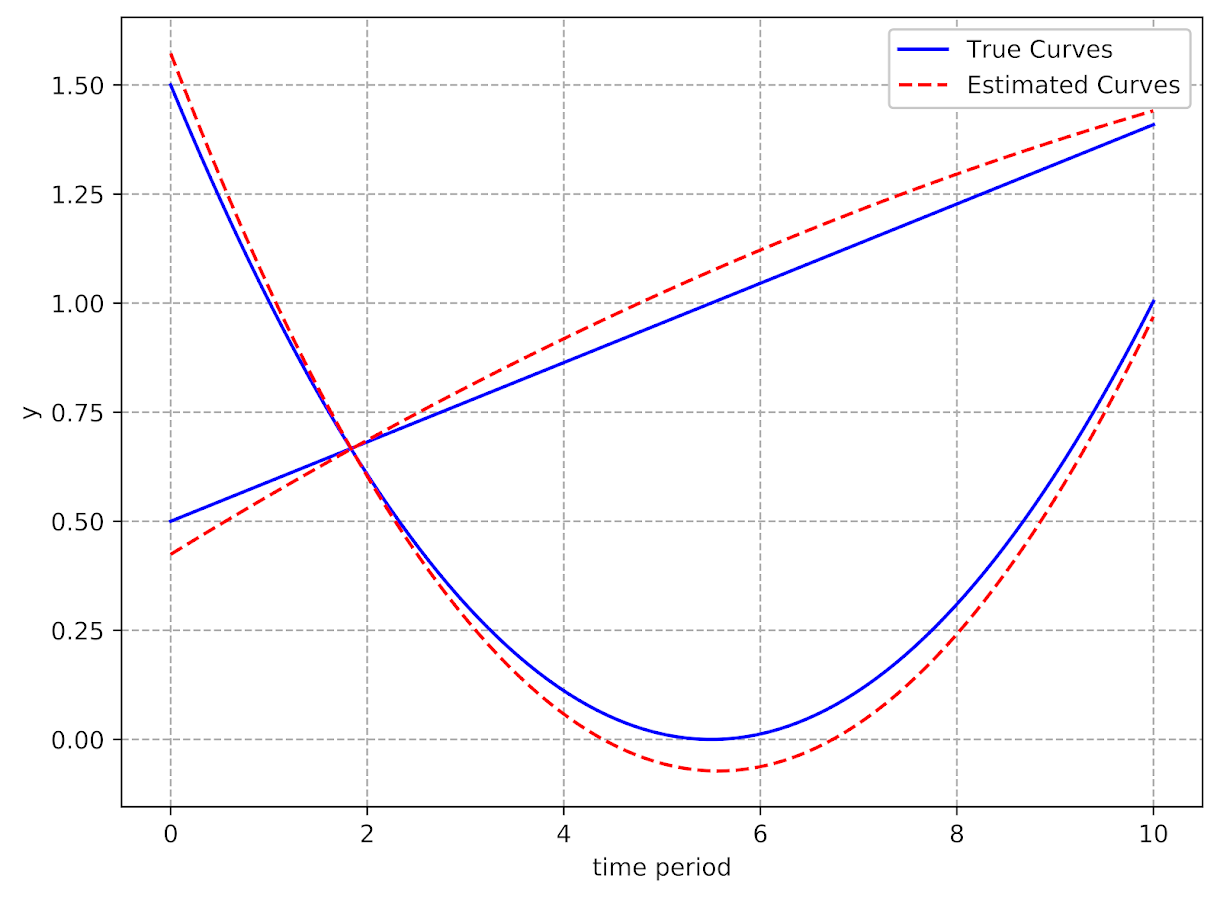It took quite a few iterations to converge though, (iterations were super fast, but it is a tiny problem, so not sure how timing will generalize) and only converged when using the Adam optimizer (stochastic gradient descent converged to an answer with a similar mean square error, but not to anywhere near the right answer). These models are notorious for converging to sub-optimal locations, so that may just be an intrinsic part of the problem and a good library needs to do better with starting conditions.

I have a few notes about potential updates to the code at the end of my Jupyter notebook. For count or binomial 0/1 data, that should be a pretty easy update. Also need to write code to do out of sample predictions (which I think I can figure out as well). A harder problem I am not sure how to figure out is to do an equation for the latent groups inside of the function. And I don’t know how to get standard errors for the coefficient estimates. Hopefully I can figure that out while trying to teach myself some more deep learning. I have a few convolution ideas I want to try out for spatial-temporal crime forecasting and include proactive police feedback, but I won’t get around to them for quite awhile I imagine.